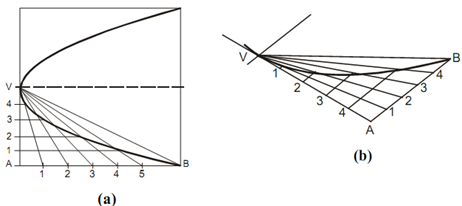## Construction of Parabola via Parallelogram Method Assignment Help

Assignment Help: >> Parabola - Construction of Parabola via Parallelogram Method

Construction of Parabola via Parallelogram Method:

As the dimensions for a parallelogram that will enclose a given parabola are normally recognized, a parabola may be constructed via the parallelogram method like explained in diagram. Along with the enclosing rectangle drawn to the given dimensions, divide VA and AB into the similar number of equal divisions or five here and number the division points like shown. Sketch lines from point V to each of the division point along AB, that is V1, V2, V3 and V4. Then sketch lines parallel to the axis from points 1, 2, 3 and 4 on VA. The intersection of the lines via 1 along with V1, 2 along with V2, 3 along with V3 and 4 along with V4 are on the parabolic curve. Connect them and draw parabolic curve. The method as explained for a parabola put in a rectangle may be applied to a non-rectangular shape as displayed in diagram (b).